• 11 years 16 weeks ago

It may be better to use "contour integral" here rather than "path integral" - I think the latter is more often used for the integrals in quantum field theory than in complex analysis.

Also, there's something odd with the use of the "Add" tag at the end of the general discussion that I don't see how to easily fix.

• 11 years 16 weeks ago

I renamed it (and added another example, while I'm here...)

• 11 years 16 weeks ago Elementary number theory front page

That's a nice one. Another example might be a proof of the irrationality of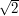or maybe a proof that the diophantine equation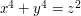has no positive integral solutions.

• 11 years 16 weeks ago Elementary number theory front page

Definitely agreed. A nice example would be the infinite-descent proof that every positive integer is a sum of four squares.

• 11 years 16 weeks ago

Vicky, if you spot more of those it would be great. I've just found one of my own: using the law of trichotomy. I'm about to change it to something more descriptive and imperative.

• 11 years 16 weeks ago Elementary number theory front page

I believe Fermat's 'descente infinie' deserves a mention here. What's your opinion?

• 11 years 16 weeks ago

• 11 years 16 weeks ago

Obviously that proof is not appropriate in this article. But it might be nice to write an account of it, decide what general trick it illustrates, and write a geometry article about that general trick. Then one could link from this article saying that there was an alternative, more conceptual proof.

• 11 years 16 weeks ago

An even better approach might be to take the Riemann sphere point of view.It is then natural that the Mobius transformations are the rotations of this sphere and the preservation of circles follows from the fact that stereographic projections map circles to circles.

• 11 years 16 weeks ago

One other thing is that in an article I wrote on group presentations I gave two proofs that the group with two generatorsandand the relations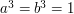is infinite. One was a direct attack and the other was to find an action on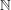with an infinite orbit. It's similar enough that some kind of cross-linking might be good (or alternatively one might just repeat the example here – my view is that it's fine if the same example appears in more than one article). I won't do anything yet, given that the structure of this article is not yet clear.

• 11 years 16 weeks ago

Yes. BS(1,2) is isomorphic to the semidirect product of Q_2 and Z, where Z acts via multiplication by 2. Which gives yet another way to see do it, by considering actions on pairs of these things.

I rather like the idea of including all these different approaches. There are interesting stories to be told about which generalize to other, similar, groups. For instance, Tim's nice observation works because BS(1,2) is residually finite. But BS(2,3) isn't, so it won't work there.

• 11 years 16 weeks ago

One can map a into the translation x -> x+1, and b to the dilation x -> x/2, and it's looking like this is a faithful representation. Well, it's still a good example of the general principle, at least...

• 11 years 16 weeks ago

More generally, I guess one can go look for nontrivial matriceswhich are conjugate to their square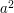(not hard to guess such matrices, after thinking a little about what their eigenvalues must be like), and that gives a whole bunch of useful representations. Easy in hindsight :-) [and, annoyingly enough, I actually knew this; this is how one proves the Frobenius lemma that the irreducible representations of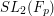are large. I should have known better than to try to attack the word problem directly...]

I'm not sure how to structure the article now; I feel that my pedestrian way of doing things is instructive, if perhaps only in the negative sense of showing what not to do. Perhaps we can give several different proofs of the same result?

I didn't know about Britton's lemma, so I learned something today!

• 11 years 16 weeks ago

Isn't the relation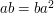satisfied in the dihedral group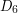? In other words, if we add the relations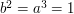, we get a group where we know thatandare non-trivial (e.g. because it acts faithfully on a triangle). So there's our homomorphism. Or am I missing something?

• 11 years 16 weeks ago

Well, there's a theory for dealing with this. When you use it, then things are certainly simpler!

The group you're considering here is the Baumslag–Solitar group BS(1,2). The standard way to prove thatis non-trivial is to observe that the group is an HNN extension of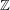and appeal to Britton's Lemma. You're basically reproving Britton's Lemma in this case.

Another (morally equivalent) point of view is to say that the object on which your group acts is the universal cover of a space constructed by gluing one end of a cylinder to itself via a 2-to-1 covering map. The fact that the circle in the cylinder lifts to a line in the universal cover showshas infinite order.

I'd be tempted to do an example like the (2,3,7)-triangle group, and construct the action on the hyperbolic plane.

Perhaps we can segue this article into a discussion of Britton's Lemma, graphs of groups etc. Hmmm.

• 11 years 16 weeks ago Complete the square

Hmm, I thought this was a common term, but I just googled for it and the first mathematical occurrence of it was... one of my own web pages. But "shifting the contour" is a reasonably common term. It's not _quite_ the same as complex substitution (it's based on Cauchy's theorem for contour integrals, rather than the change of variables formula), but it of course combines very well with such substitutions. But it also can be used independently of complex substitution, e.g. to evaluate integrals such as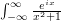by shifting the contour to a big semicircle etc.

I link to this nonexistent article in a couple other places, so I think I'll start a stub on it, and perhaps return to it later.

• 11 years 16 weeks ago Look at small cases

Maybe you are right. There are loads of problems that can be solved by doing specific cases only. Lucas's identity in elementary number theory might be a good example.(Adding the terms in the Pascal's triangle diagonally yields the Fibonacci numbers)

• 11 years 16 weeks ago Look at small cases

I know I suggested putting this example here, but now I look at it carefully, I wonder whether it really is a prime example of Gel'fond's advice. It seems to me that the basic proof (even if you dress it up with matrices) is this: expand out both sides and observe that you get the same answer. And it's not clear to me that doing that for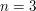is any easier than doing it for the general case.

A truly convincing example of this trick is one where the only realistic way of doing the general case is to do small cases and spot a non-obvious pattern.

• 11 years 16 weeks ago Look at small cases

I have added the example mentioned above. It might still require some editing though. You may state some prerequisites for the above example but I personally think that it's fairly straightforward and that elementary linear algebra would suffice.

• 11 years 16 weeks ago

The above example is arguably an instance of a more general discretization strategy: that of replacing compact spaces by finite complexes. For example, every compact manifold has the homotopy type of (and hence the same cohomology as) a finite CW complex. Perhaps more in the spirit of this article, every compact subset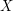of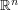can be written as the intersection of a decreasing sequence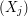of compact sets, each of which is a finite simplicial complex. (Just take successive triangulations and keep the bits that intersect.) One can hope to use simple arguments (such as induction on the number of simplices) to deduce some property about the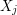, and then use a limiting argument to deduce the property for. For example, the C*-algebra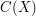of continuous functions onis the direct limit of the algebras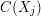. If one is, say, interested in proving something about the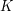-theory of, then the relevant continuity property is that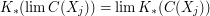(and a useful observation might be that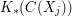is finitely generated).

• 11 years 17 weeks ago Complete the square

I've started an article about making complex substitutions in integrals. My guess is that this is what you mean by contour shifting, but I have not put this link in yet because I am not quite sure. If it is, and if this is the standard name for the technique, then I'll add a remark to that effect to the article.

• 11 years 17 weeks ago Complete the square

I put up some quick examples to reflect them. Feel free to edit, of course.

• Anonymous (not verified) 11 years 17 weeks ago Complete the square

I've seen this used multiple times, in a context similar to the usual proof of Cauchy-Schwarz inequality, where completing the square of a real-valued expression shows that some grouping of terms is non-negative.

• 11 years 17 weeks ago Look at small cases

Absolutely.There should be a string of articles.Hopefully, many more articles would come up with an explanation.Tricky is going to be a great ride.

• 11 years 17 weeks ago Look at small cases

I marked it as a stub because it had neither a "General discussion" section nor any examples. It is certainly interesting and valuable advice, but doesn't quite count as "mathematically interesting information" in the intended sense, because it doesn't yet contain any actual mathematics. But if, say, you were to add the example that is explained on the page you suggest, then it would instantly lose its stub status.

Just to repeat, it is possible for a Tricki article to contain something interesting, but still to count as a stub. So you should not take the stub designation as in any way suggesting that Gel'fond's suggestion is uninteresting. But I would see the quotation as the beginning of what should ultimately be a quite long article with several examples: a principle this important deserves nothing less.